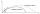Vector + triangle - examples

1. TriangleTriangle KLM is given by plane coordinates of vertices: K[-12, -11] L[-15, -18] M[-13, -12]. Calculate its area and itsinterior angles.
2. Two forcesTwo forces with magnitudes of 25 and 30 pounds act on an object at angles of 10° and 100° respectively. Find the direction and magnitude of the resultant force. Round to two decimal places in all intermediate steps and in your final answer.An airplane leaves an airport and flies due west 120 miles and then 150 miles in the direction S 44.1°W. How far is the plane from the airport (round to the nearest mile)?
4. Vector sumThe magnitude of the vector u is 12 and the magnitude of the vector v is 8. Angle between vectors is 61°. What is the magnitude of the vector u + v?A ship travels 84 km on a bearing of 17°, and then travels on a bearing of 107° for 135 km. Find the distance of the end of the trip from the starting point, to the nearest kilometer.
6. Three vectorsThe three forces whose amplitudes are in ratio 9:10:17 act in the plane at one point so that they are in balance. Determine the angles of the each two forces.
7. BearingA plane flew 50 km on a bearing 63°20' and the flew on a bearing 153°20' for 140km. Find the distance between the starting point and the ending point.
8. Medians and sidesTriangle ABC in the plane Oxy; are the coordinates of the points: A = 2.7 B = -4.3 C-6-1 Try calculate lengths of all medians and all sides.
9. Ballistic curveThe ballistic grenade was fired at a 45° angle. The first half ascended, the second fall. How far and how far it reached if his average speed was 1200km/h, and 12s took from the shot to impact.
10. ForcesForces with magnitudes F1 = 42N and F2 = 35N act at a common point and make an angle of 77°12'. How big is their resultant?
11. Two forcesThe two forces F1 = 580N and F2 = 630N have the angle of 59 degrees. Calculate their resultant force F.

We apologize, but in this category are not a lot of examples.
Do you have an interesting mathematical example that you can't solve it? Enter it, and we can try to solve it.

To this e-mail address, we will reply solution; solved examples are also published here. Please enter e-mail correctly and check whether you don't have a full mailbox.

Two vectors given by its magnitudes and by included angle can be added by our vector sum calculator. See also our trigonometric triangle calculator.